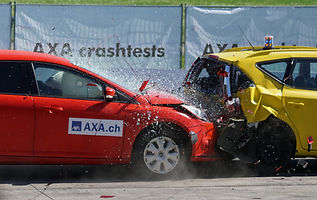top of page

### PHYSICS: 1st Semester Modules

Each curriculum module begins with a description of an interesting phenomenon or problem that drives the lessons. The relevant physics is introduced to explore the phenomenon or problem. The curriculum emphasizes the development of mathematical models that can be used to describe,and understand these systems. Each module includes short readings for students, tutorials, activities, and case studies.##### ​

This modules explores motion, velocity, and acceleration through the lens of video analysis. Students take and analyze videos of simple motions to build mathematical models for motion. This helps students to connect definitions and equations to graphs, and builds to a discovery of the kinematics equations.##### ​

In this module, students develop an understanding of energy and energy conservation through the study of alternative energy. Lessons focus on wind power (kinetic energy), hydropower (gravitational potential energy), and nuclear energy. Also included is a case study of the Belo Monte Dam in Brazil, which explores sociocultural issues related to alternative energy. The final project is a "shark tank" style pitch to the class.##### ​

Newton's Laws and Momentum Conservation are introduced as mathematical models to understand motion and collisions. The final project requires students to use data from a car accident to determine who was at fault in a collision. They submit an expert witness testimony, such as might be used in court.##### ​

In this module, students apply their understanding of forces and learn about statics (torque, stress, strain) by studying bridge design and bridge failures. For the final project, students construct a bridge, test it to failure, and analyze the failure mathematically.##### ​

Gravitation, circular motion, and rotational motion are introduced in this unit through the lens of astronomy and space travel.  Tutorials explore how the historical models of the solar system developed and how Newton's Law of Gravity can be applied to launch spacecraft. In the final project, students use a Python simulation to launch a spacecraft to a designated body in the solar system.

bottom of page